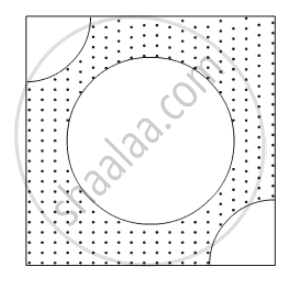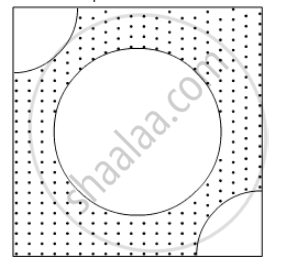Advertisement Remove all ads

# From Each of the Two Opposite Corners of a Square of Side 8 Cm, a Quadrant of a Circle of Radius 1.4 Cm is Cut. Another Circle of Radius 4.2 Cm is Also Cut from the Centre - Mathematics

Sum

From each of the two opposite corners of a square of side 8 cm, a quadrant of a circle of radius 1.4 cm is cut. Another circle of radius 4.2 cm is also cut from the centre as shown in the following figure. Find the area of the remaining (Shaded) portion of the square. (Use π = 22/7)Advertisement Remove all ads

#### Solution

It is given that a circle of radius 4.2 cm and two quadrants of radius 1.4 cm are cut from a square of side 8 cm.Let the side of square be a. Then,

Area of squre=a^2

=8xx8

=64 cm^2

$Area of circle = \pi r^2$
$= \frac{22}{7} \times 4 . 2 \times 4 . 2$
$= 55 . 44 {cm}^2$

Now area of quadrant of circle of radius 1.4 cm is,

Area of quadrant =1/4pir^2

  =1/4xx22/7xx1.4xx1.4

=1.54 cm^2

$Area of circle = \pi r^2$
$= \frac{22}{7} \times 4 . 2 \times 4 . 2$
$= 55 . 44 {cm}^2$

Is there an error in this question or solution?
Advertisement Remove all ads

#### APPEARS IN

RD Sharma Class 10 Maths
Chapter 13 Areas Related to Circles
Exercise 13.4 | Q 14 | Page 57
Advertisement Remove all ads

#### Video TutorialsVIEW ALL 

Advertisement Remove all ads
Share
Notifications

View all notifications

Forgot password?
Course